## Welcome to the Second Life Forums Archive

These forums are CLOSED. Please visit the new forums HERE

# llGetTimestamp() <-> unix timestamp

Masakazu Kojima
ケロ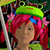Join date: 23 Apr 2004
Posts: 232
02-18-2005 07:39
This code converts a timestamp as returned by llGetTimestamp() to a unix timestamp, ie, an integer repesenting the number of seconds since 0 hours, 0 minutes, 0 seconds, January 1, 1970 UTC. This is the same format as the time parameter in the email() event. It can also convert a unix timestamp back to the llGetTimestamp() format (without the float portion).

There are also some not very good unit test functions.

I don't know if something like this has been posted before. I found something in an old thread, but it failed all of my tests.

This code is public domain.

CODE
`// vvvvvvvvvvvvvvvvvvvvvv// * UNIXTIME FUNCTIONS *// vvvvvvvvvvvvvvvvvvvvvv// Return the number of seconds since 0 hours, 0 minutes, 0 seconds, January 1, 1970 UTCinteger timestamp_to_unixtime(string timestamp) {    // YYYY-MM-DDThh:mm:ss.ff..fZ    // 0123456789012345678    // llGetSubString is slow, but in testing is faster than using llParseStringToList    // 100 iterations using llGetSubstring averaged 6 seconds, llParseStringToList took 9.    integer year    = (integer) llGetSubString(timestamp,  0,  3);    integer month   = (integer) llGetSubString(timestamp,  5,  6);    integer day     = (integer) llGetSubString(timestamp,  8,  9);    integer hour    = (integer) llGetSubString(timestamp, 11, 12);    integer minute  = (integer) llGetSubString(timestamp, 14, 15);    integer second  = (integer) llGetSubString(timestamp, 17, 18);    integer days    = 0;    integer time    = 0;    integer i;        days += (year - 1970) * 365;    // Number of leap years that have passed since 1970 is floor( ((year - 1) - 1968) / 4 ),    // since the current year hasn't passed (-1) and 1972 is the first leap year after 1970 (-1968).    // For example, 2004 - 1 - 1968 is 35, and 35 / 4 is 8.75, floor(8.75) is 8    // 1972, 1976, 1980, 1984, 1988, 1992, 1996, 2000, 2004    //   1     2     3     4     5     6     7     8     9    // 2005 - 1 - 1968 is 36, 36 / 4 is 9    //    // To be more correct, years divisible by 100 aren't leap years unless they are also divisible by    // 400, but the first year after 1970 that won't really be a leap year is 2100, and the format will    // overflow by 2038.    // Casting to integer has the same effect as llFloor(), but it is faster.    days += (integer) (((year - 1) - 1968) / 4);    if ( month > 1 ) days += 31;    if ( month > 2 ) days += 28 + ((year % 4) == 0);    if ( month > 3 ) days += 31;    if ( month > 4 ) days += 30;    if ( month > 5 ) days += 31;    if ( month > 6 ) days += 30;    if ( month > 7 ) days += 31;    if ( month > 8 ) days += 31;    if ( month > 9 ) days += 30;    if ( month > 10 ) days += 31;    if ( month > 11 ) days += 30;        days += day - 1;        // - 1 because the current day hasn't passed yet.                    time += days   * 86400; // SECONDS_PER_DAY;    time += hour   * 3600;  // SECONDS_PER_HOUR;    time += minute * 60;    // SECONDS_PER_MINUTE;    time += second;    return time;   }string unixtime_to_timestamp(integer unixtime) {    integer year;    integer month;    integer day;    integer hour;    integer minute;    integer second;    string  ds;    string  ts;    integer day_temp;        second  = unixtime;    day     = (integer) second / 86400;    second %= 86400;    hour    = (integer) second / 3600;    second %= 3600;    minute  = (integer) second / 60;    second %= 60;        // caveat: does not check to see if leap days have made an extra year    year = 1970;    while ( day >= 365 ) {        day -= 365 + ((year % 4) == 0);        year += 1;    }    //year    = llFloor(day / 365) + 1970;    //day     = (day % 365) + llFloor(((year - 1968) - 2) / 4) + 1;        // forget about leap day for the moment    day_temp = day - ((day >= 59) && ((year % 4) == 0));//    llWhisper( 0, (string) day_temp + " / " + (string) day ) ;             if ( day_temp >= 334 ) { month = 12; day_temp -= 334; } // 30    else if ( day_temp >= 304 ) { month = 11; day_temp -= 304; } // 31    else if ( day_temp >= 273 ) { month = 10; day_temp -= 273; } // 30    else if ( day_temp >= 243 ) { month =  9; day_temp -= 243; } // 31    else if ( day_temp >= 212 ) { month =  8; day_temp -= 212; } // 31    else if ( day_temp >= 181 ) { month =  7; day_temp -= 181; } // 30    else if ( day_temp >= 151 ) { month =  6; day_temp -= 151; } // 31    else if ( day_temp >= 120 ) { month =  5; day_temp -= 120; } // 30    else if ( day_temp >=  90 ) { month =  4; day_temp -=  90; } // 31    else if ( day_temp >=  59 ) { month =  3; day_temp -=  59; } // 28    else if ( day_temp >=  31 ) { month =  2; day_temp -=  31; } // 31    else                        { month =  1;                  }    day = day_temp + 1 + ((day == 59) && ((year % 4) == 0));     //2147483647    //  YYYYMMDD    //  01234567    //    HHMMSS    //    012345        ds = (string) ((year * 10000) + (month * 100) + day);    ts = (string) ((hour * 10000) + (minute * 100) + second);    while ( llStringLength(ts) < 6 ) ts = "0" + ts;        return llGetSubString(ds, 0, 3) + "-" + llGetSubString(ds, 4, 5) + "-" + llGetSubString(ds, 6,7) + "T" +           llGetSubString(ts, 0, 1) + ":" + llGetSubString(ts, 2, 3) + ":" + llGetSubString(ts, 4,5) + "Z";    }// ^^^^^^^^^^^^^^^^^^^^^^// * UNIXTIME FUNCTIONS *// ^^^^^^^^^^^^^^^^^^^^^^// vvvvvvvvvvvvvvvvvvvvvv// * UNITTEST FUNCTIONS *// vvvvvvvvvvvvvvvvvvvvvvinteger assertions;integer assertions_passed;assert_equal_string(string str1, string str2) {    assertions += 1;    if ( str1 != str2 ) llWhisper( 0, "ASSERTION FAILED: assert_equal_string(\"" + str1 + "\", \"" + str2 + "\")");    else assertions_passed += 1;}assert_equal_integer(integer i1, integer i2) {    assertions += 1;    if ( i1 != i2 ) llWhisper( 0, "ASSERTION FAILED: assert_equal_integer(" + (string)i1 + ", " + (string)i2 + ")");    else assertions_passed += 1;}report() {    llWhisper(0, "Assertions passed: " + (string)assertions_passed + "/" + (string)assertions );}// ^^^^^^^^^^^^^^^^^^^^^^// * UNITTEST FUNCTIONS *// ^^^^^^^^^^^^^^^^^^^^^^                                                default {    state_entry() {        // timestamps to test against taken from the `date` command on a FreeBSD machine        // eg: date -j -f "%FT%TZ" "2005-01-30T21:33:11Z" "+%s"        assert_equal_integer( 0, timestamp_to_unixtime( "1970-01-01T00:00:00.000000Z" ) );          assert_equal_string( "1970-01-01T00:00:00Z", unixtime_to_timestamp( timestamp_to_unixtime( "1970-01-01T00:00:00Z" ) ) );        assert_equal_integer( 31536000, timestamp_to_unixtime( "1971-01-01T00:00:00.000000Z" ) );          assert_equal_integer( 94694400, timestamp_to_unixtime( "1973-01-01T00:00:00.000000Z" ) );                 assert_equal_string( "2005-01-30T21:33:11Z", unixtime_to_timestamp( 1107120791 ) );        assert_equal_string( "2001-03-04T00:01:37Z", unixtime_to_timestamp( 983664097 ) );        assert_equal_integer( 951743049, timestamp_to_unixtime( "2000-02-28T13:04:09Z" ) );        assert_equal_string( "2000-02-28T13:04:09Z", unixtime_to_timestamp( 951743049 ) );        assert_equal_string( "2000-02-29T12:34:56Z", unixtime_to_timestamp( timestamp_to_unixtime( "2000-02-29T12:34:56" ) ) );        assert_equal_integer( 951916448, timestamp_to_unixtime( "2000-03-01T13:14:08Z" ) );        assert_equal_string( "2000-03-01T13:14:08Z", unixtime_to_timestamp( 951916448 ) );        assert_equal_string( "2001-02-28T12:34:56Z", unixtime_to_timestamp( timestamp_to_unixtime( "2001-02-28T12:34:56" ) ) );                //string ts = llGetTimestamp();        //llWhisper( 0, ts + " = " + unixtime_to_timestamp( timestamp_to_unixtime( ts ) ) );                report();    }}`
Francis Chung
This sentence no verb.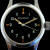Join date: 22 Sep 2003
Posts: 918
03-07-2005 07:13
Good show Masakazu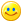I needed a high-precision, potentially long-lived timer for one of my projects, so this came in very handy.

I added code to differentiate the amount of time (in seconds) between two timestamps, and performance is very important in my application so I optimized the timestamp_to_unixtime function a little by using an index lookup instead of repeated comparison/addition. It's roughly 5-20% faster in LSL from my tests.

Thanks!

CODE
`// timeStamp2 - timeStamp1 in secondsfloat diffTimestamps( string timeStamp1, string timeStamp2 ) {    float diff = (timestamp_to_microseconds(timeStamp2) - timestamp_to_microseconds(timeStamp1)) / 1000000.0;    return diff + (timestamp_to_unixtime(timeStamp2) - timestamp_to_unixtime(timeStamp1));}// Returns the fractional part of the timestampinteger timestamp_to_microseconds(string timestamp) {    return (integer) llGetSubString(timestamp,  20, 25);}// This function based on Code posted by Masakazu Kojimalist daysPerMonth = [ 0, -1,  30, 58, 89, 119, 150, 180,  211, 242, 272, 303, 333 ];integer timestamp_to_unixtime(string timestamp) {    // YYYY-MM-DDThh:mm:ss.ff..fZ    // 0123456789012345678    // llGetSubString is slow, but in testing is faster than using llParseStringToList    // 100 iterations using llGetSubstring averaged 6 seconds, llParseStringToList took 9.    integer year    = (integer) llGetSubString(timestamp,  0,  3);    integer month   = (integer) llGetSubString(timestamp,  5,  6);    integer day     = (integer) llGetSubString(timestamp,  8,  9);    integer hour    = (integer) llGetSubString(timestamp, 11, 12);    integer minute  = (integer) llGetSubString(timestamp, 14, 15);    integer second  = (integer) llGetSubString(timestamp, 17, 18);    integer days    = 0;    integer time    = 0;    integer i;        days += (year - 1970) * 365;    // Number of leap years that have passed since 1970 is floor( ((year - 1) - 1968) / 4 ),    // since the current year hasn't passed (-1) and 1972 is the first leap year after 1970 (-1968).    // For example, 2004 - 1 - 1968 is 35, and 35 / 4 is 8.75, floor(8.75) is 8    // 1972, 1976, 1980, 1984, 1988, 1992, 1996, 2000, 2004    //   1     2     3     4     5     6     7     8     9    // 2005 - 1 - 1968 is 36, 36 / 4 is 9    //    // To be more correct, years divisible by 100 aren't leap years unless they are also divisible by    // 400, but the first year after 1970 that won't really be a leap year is 2100, and the format will    // overflow by 2038.    // Casting to integer has the same effect as llFloor(), but it is faster.    days += (integer) (((year - 1) - 1968) / 4);        // Get the number of days throughout the year    days += llList2Integer( daysPerMonth, month );    days += day;        // And account for if current year is a leap year    if ( month > 2 ) days+= !(year % 4);        time += days   * 86400; // SECONDS_PER_DAY;    time += hour   * 3600;  // SECONDS_PER_HOUR;    time += minute * 60;    // SECONDS_PER_MINUTE;    time += second;    return time;   }`
_____________________
--
~If you lived here, you would be home by now~
Strife Onizuka
Moonchild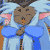Join date: 3 Mar 2004
Posts: 5,887
03-07-2005 19:19
CODE
`    days += (integer) (((year - 1) - 1968) / 4);`

can be replaced with

CODE
`    days += (year - 1969) / 4;`

your numbers are already integers so you don't need to to type cast them. Should shave off maybe a second off a thousand iterations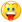basicly if you don't have any floats in your code, none of the functions return floats, then all your math is integer math. You will never have to type cast them to integers.

but if you want this to run fast you need to simplify, less code runs faster then more code.
CODE
`integer year    = (integer) llGetSubString(timestamp,  0,  3);integer month   = (integer) llGetSubString(timestamp,  5,  6);integer time    = 0;return  (   (year * 365) + ((year - 2878169) / 4) +             llList2Integer( daysPerMonth, month ) +             (integer) llGetSubString(timestamp,  8,  9) +            ((month>2) && !(year % 4))        ) * 86400 +         (integer)llGetSubString(timestamp, 11, 12) * 3600 +         (integer)llGetSubString(timestamp, 14, 15) * 60 +         (integer)llGetSubString(timestamp, 17, 18)`

to conclude:
32 bit floats suck.

EDIT:
Sorry about the math error in the leap year,
was: (year / 4) - 719542
now is: ((year - 2878169) / 4)
_____________________
Truth is a river that is always splitting up into arms that reunite. Islanded between the arms, the inhabitants argue for a lifetime as to which is the main river.
- Cyril Connolly

Without the political will to find common ground, the continual friction of tactic and counter tactic, only creates suspicion and hatred and vengeance, and perpetuates the cycle of violence.
- James Nachtwey
Masakazu Kojima
ケロJoin date: 23 Apr 2004
Posts: 232
03-09-2005 14:27
I decided to play around with it and see if I could make it even faster. Here is what I came up with, comments from the original omitted:

CODE
`list DAYS_PER_MONTH = [ 0, -1,  30, 58, 89, 119, 150, 180,  211, 242, 272, 303, 333 ];integer timestamp_to_unixtime(string timestamp) {    integer year    = (integer) llGetSubString(timestamp,  0,  3);    integer month   = (integer) llGetSubString(timestamp,  5,  6);        return    (        // days = (year - 1970) * 365;        (year - 1970) * 365        // days += (integer) (((year - 1) - 1968) / 4);        + (year - 1969) / 4        // days += llList2Integer( daysPerMonth, month );        + llList2Integer( DAYS_PER_MONTH, month )        // days += day;        + (integer) llGetSubString(timestamp,  8,  9)        // if ( month > 2 ) days+= !(year % 4);        + (month>2) * !(year % 4)    )    // time += days   * 86400;    * 86400    // time += hour   * 3600;  // SECONDS_PER_HOUR;    + (integer) llGetSubString(timestamp, 11, 12) * 3600    // time += hour   * 3600;  // SECONDS_PER_HOUR;    + (integer) llGetSubString(timestamp, 14, 15) * 60    // time += second;    + (integer) llGetSubString(timestamp, 17, 18);}`

100 iterations of the 5 timestamp_to_unixtime tests above (all 3 started at the same time):
unixtime 1.0.2 whispers: 65.830215
unixtime 1.0.2 whispers: Assertions passed: 500/500
unixtime 1.0.1-francis whispers: 87.438416
unixtime 1.0.1-francis whispers: Assertions passed: 500/500
unixtime 1.0.0 whispers: 95.783142
unixtime 1.0.0 whispers: Assertions passed: 500/500
Francis Chung
This sentence no verb.Join date: 22 Sep 2003
Posts: 918
03-10-2005 18:48
Good stuffI didn't think an integer->integer cast would make a difference, since there's no operation there. So I ran a test.

Surprisingly, it did! 1000 integer->integer casts costs you roughly between .05 to .4 seconds.

I didn't want to compact everything down to one line of code, for readability. But I like Masakazu's code. Still one line, but very readable. I've backported it into my project_____________________
--
~If you lived here, you would be home by now~